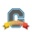cancel
Showing results for
Did you mean:I am wondering if anyone else would like to see a differentiation between questions actually answered and those questions that are automatically marked assumed answered?

I Just marked one of my questions assumed answered (and I have seen other questions marked assumed answered - probably due to lack of activity).  I marked mine assumed answered because a work around was provided, and it was provided under the older community; however, the actual issue is not answered.  In my case, it should probably be written up as a feature request (or feature fix).

I can see the case where questions are marked as assumed answer when in reality the answer has not been supplied yet.

Tags (2)
1 Solution

Accepted SolutionsCommunity Member

@bowmanr ​, sorry for the delayed response. I can see ways 'assumed' and 'correct' answers might appear confusing. In short, assumed answered is when the right answer or multiple correct answers (perhaps work-around solutions or several options) are included in the conversation thread. The question where you marked 'assumed correct' is a great example of that.

Correct answer is when there is a much clearer solution or a response that surfaces as the authoritative answer -- for instance from a Coach, a Community Manager or someone from the product team.

Either way, when you search through all questions and filter by "marked correct" you will receive a list that includes both assumed and correct answers.

Hope this helps clarify!

And, of course, I'm officially marking this answer correct2 RepliesCommunity Coach

@bowmanr ​...

Since this question seems to be more about the Jive interface (which is what the 2.0 Canvas Community runs on), I'm going to move this question over to the Meta Community Group​ where Instructure is housing these kinds of questions.Community Member

@bowmanr ​, sorry for the delayed response. I can see ways 'assumed' and 'correct' answers might appear confusing. In short, assumed answered is when the right answer or multiple correct answers (perhaps work-around solutions or several options) are included in the conversation thread. The question where you marked 'assumed correct' is a great example of that.

Correct answer is when there is a much clearer solution or a response that surfaces as the authoritative answer -- for instance from a Coach, a Community Manager or someone from the product team.

Either way, when you search through all questions and filter by "marked correct" you will receive a list that includes both assumed and correct answers.

Hope this helps clarify!

And, of course, I'm officially marking this answer correct